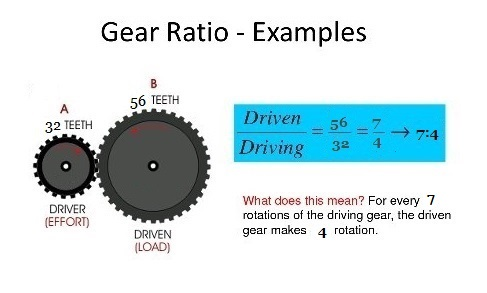## Monday, 25 December 2017

### GEAR RATIO | SPEED RATIOImage Courtesy : Google
The gear ratio is also necessarry to consider when selcting the gears for any system. The larger the gear ratio, the greater the difference in gear speed and torque. The ratio of gear train is defined as, "it is the ratio of the angular velocity of the driver (Input gear) to the angular velocity of driven gear (Output gear)", it is alo called speed ratio of gear train. The gear ratio can be computed directlly from the number of number of teeth of the various gears that engauge to form the gear train.
Gear ratio is found by "dividing the number of teeth in the output gear (gear being driven) by the number of teeth in the driving gear". In simple words, gear ratio defines the relationhip between the number of teeth of multiple gears.
Gear Ratio = Output gear #teeth/ Input Gear # teethImage Courtesy : Google
For example, consider a motor is connected to a gear A with 70 number of teeth and this gear is then connected to anothe gear B with 40 number of teeth, that drives a wheel, then our gear ratio is 70:40 or more accurately 7:4.
In the above example, if Gear A was driving the system, the gear ratio would be 7/4. If Gear B was driving the system the gear ratio would be 4/7.
If you can't count the gear teeth (or if they do not exist), gear ratio's may also be calculated by measuring the distance between the center of every gear to the point of contact.
For example, if a motor is connected to a gear with 1" (1 inch) diameter and this gear is connected to the a gear with 2" diameter connected to a wheel.

From the center to edge of our input gear is 0.5"
from the center to edge of our output gear is 1"
Our gear ratio is 1/0.5 or more accurately 2:1.Image Courtesy : Google